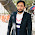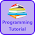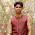# Programming Videos

## Search

### Binary Search Program Using Recursion in C, C++

Write a C, C++ code to implement binary search program using recursion.

What is Binary Search?

Binary Search algorithm is used to search an element in a sorted array. Binary search works by comparing the value to the middle element of an array. If the value is found then index is returned otherwise the steps is repeated until the value is found.

It is faster than linear search.

Time complexity of Linear search is O(n).

Time complexity of Binary search is O(log(n)).

What is Recursion?

In recursion, the function call itself until the base condition is reached.  If you are not familiar with recursion then check the difference between recursion and iteration.

Reverse a number using recursion.

In my previous post we have implemented binary search using iterative approach. In this post. we are going to implement binary search using recursion.

## Implement Binary Search Using Recursion in C

```#include <stdio.h>
#include <stdlib.h>

void BinarySearch(int arr[],int num,int first,int last){

int mid;

if(first > last){

} else {

/* Calculate mid element */
mid = (first + last)/2;

/* If mid is equal to number we are searching */

if(arr[mid]==num){

printf("Element is found at index %d ",mid);
exit(0);

}else if(arr[mid] > num){

BinarySearch(arr, num, first, mid-1);

}else{

BinarySearch(arr, num, mid+1, last);
}
}
}

main(){

int arr,beg,mid,end,i,n,num;

printf("Enter the size of an array ");
scanf("%d",&n);

printf("Enter the values in sorted sequence \n");

for(i=0;i<n;i++)
{
scanf("%d",&arr[i]);
}

beg=0;
end=n-1;

printf("Enter a value to be search: ");
scanf("%d",&num);

BinarySearch(arr,num,beg,end);

}
```

## Implement Binary Search using Recursion in C++

```#include <iostream>
using namespace std;

int BinarySearch(int arr[], int num, int beg, int end)
{
int mid;

if (beg > end){

return 0;

} else {

mid = (beg + end) / 2;

if(arr[mid] == num){

cout << "Number is found at " << mid << " index \n";
return 0;

} else if (num > arr[mid]) {

BinarySearch (arr, num, mid+1, end);

} else if (num < arr[mid]) {

BinarySearch (arr, num, beg , mid-1);
}
}

}

int main() {

int arr, num, i, n, beg, end;

cout <<"Enter the size of an array (Max 100) \n";
cin >> n;

cout <<"Enter the sorted values \n";

for(i=0; i<n; i++) {

cin >> arr[i];
}

cout <<"Enter a value to be search \n";
cin >> num;

beg = 0;
end = n-1;

BinarySearch (arr, num, beg, end);

return 0;
}

```

Output :

```Enter the size of an array (Max 100) : 6
```
`Enter the sorted values :  2   4   6   7   8   9`
```Enter a value to be search :  7
```
`Number is found at 3 index `

1.2.3.Thanks man. I have one question does the time complexity of binary search remains same when we implement them recursively.

1.4.How could you return the correct index to pass on to another function or variable? can you keep the recursive answer when you leave the function?

5.How could you return the correct index to pass on to another function or variable? can you keep the recursive answer when you leave the function?

6.1.7.#include
using namespace std;

int search(int elem, int size, int arr[])
{

size = size - 1;
static int low = 0;
static int high = size;

int mid = (low+high)/2;

if(arr[mid] == elem)
{
return mid;
}
else if(size == 0)
{
return -1;
}
else
{
if(elem > arr[mid])
{
low = mid + 1;
search(elem, (size/2), arr);
}
else
{
high = mid - 1;
search(elem, mid, arr);
}
}
}

int main()
{
int arr[] = {10, 12, 15, 20, 25, 35, 46, 50, 80, 100};
for(int i = 0; i < 10; i++)
cout<<arr[i]<<endl;

cout<<"Element found at index: "<<search(102, 10, arr)<<endl;
return 0;
}